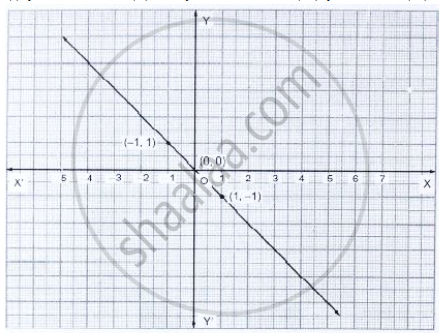# From the Choices Given Below, Choose the Equation Whose Graph is Given in Fig. Below. (I) Y = X (Ii) X + Y = 0 (Iii) Y = 2x (Iv) 2 + 3y = 7x - Mathematics

From the choices given below, choose the equation whose graph is given in Fig. below.
(i) y = x    (ii) x + y = 0    (iii) y = 2x   (iv) 2 + 3y = 7x[Hint: Clearly, (-1, 1) and (1, -1) satisfy the equation x + y = 0]

#### Solution

Clearly (-1 , 1 ) and ( 1, -1) satisfy the equation  x + y = 0
∴ The equation whose graph is given by   x + y = 0

Concept: Graph of a Linear Equation in Two Variables
Is there an error in this question or solution?

#### APPEARS IN

RD Sharma Mathematics for Class 9
Chapter 7 Linear Equations in Two Variables
Exercise 7.3 | Q 8

Share